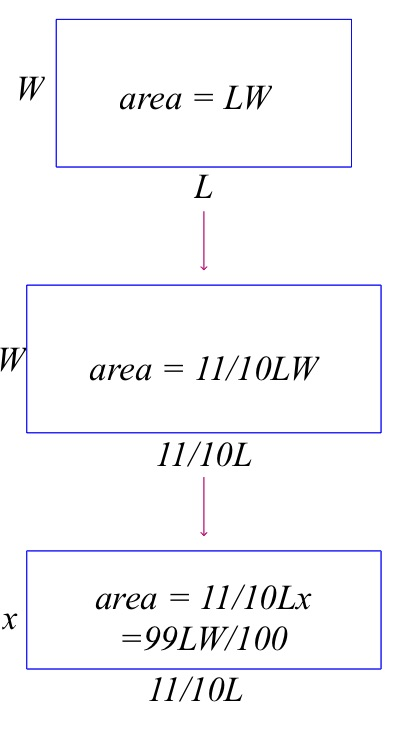# Increases and Decreases

## Problem

A rectangle has length L, width W, and area A. If L is increased by 1/10 and A is decreased by 1/100, by what fraction does W change?

## Solution

It may be tempting to say that if area decreases by 1/100 while length is increases by 1/10, then width must increase 1/100 + 1/10 = 11/100. But that doesn’t work.

Call the width of the changed triangle x.

Let’s see what’s going on:

• Area of original rectangle: .
• Length of changed rectangle: .
• Area of changed rectangle: .
• But the area of the changed rectangle also equals the changed length times the changed width: .

Set the last two expressions for the changed area A equal to each other and solve for x:

The changed width is 9/10 of the original width, so W decreases by 1/10.

Increase one dimension of a rectangle by 1/10, decrease the other by 1/10, and the result is a decrease in area of 1/100. Sound funny? Consider: When you increase the original length by 1/10, you are increasing the area by 1/10 of the original area. Then when you decrease the length by 1/10, you are decreasing the area by 1/10 of the enlarged  area. So you’ve decreased by just a little more than you increased. Hence, the net change is a very small decrease.

The same sort of thing happens when you buy an item on sale for, say, 5% off and then you have to pay 5% tax on the sale price: The final price will be just a tiny bit less than the original price without tax.